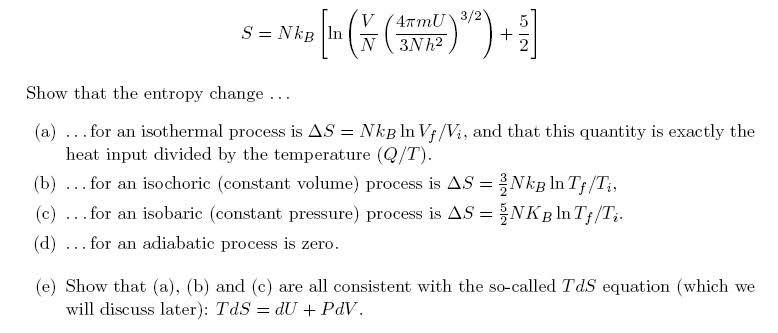# The T dS equations and Sackur-Tetrode equation

mathman44
The "T dS" equations and Sackur-Tetrode equation

## Homework Statement

This is a bit of a dumb question (I hope)I'm having trouble with part (e).

## The Attempt at a Solution

to show it for (a), dS is given, so $$T\times{NK_bln{\frac{V_f}{V_i}} = P\times{dV}$$ since dU = 0 for isothermal process. P can be written as $$\frac{NK_bT}{V}$$, but this is clearly not equal to what I got in part A... although it would be if I integrated the right side (?)

Gold Member

The TdS equation has TdS on the left hand side, you have an expression for $\Delta S$ of a process with a macroscopic change. Do you know that
$$d(\ln{x})=\frac{d(\ln{x})}{dx}dx =\frac{dx}{x}$$
?

mathman44

Was I wrong to assume that $$\Delta{S} = dS$$?

Gold Member

Yes, if you use Vf and Vi on the left hand side, you need to use them on the right hand side and take the limit
$$\lim_{V_{f}\rightarrow V_{i}}$$
on both sides and show that the ratio of the left hand side to the right hand side approaches 1, but it's much easier to just use the relation I mentioned.